Open in App
Not now

# Computer Graphics – Reflection Transformation in 3D

• Last Updated : 22 Jun, 2022

Reflection in 3D space is quite similar to the reflection in 2D space, but a single difference is there in 3D, here we have to deal with three axes (x, y, z). Reflection is nothing but a mirror image of an object.

Three kinds of Reflections are possible in 3D space:

• Reflection along the X-Y plane.
• Reflection along Y-Z plane.
• Reflection along X-Z plane.

1. Reflection along the X-Y plane: This is shown in the following figure –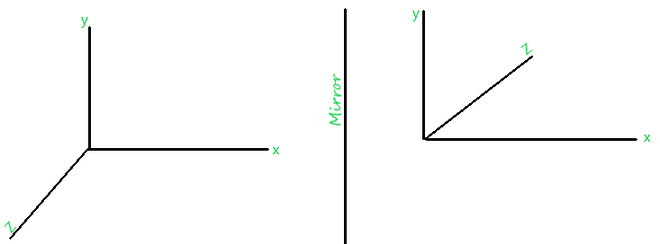Reflection along x-y plane

The Reflection transformation matrix is used to perform the reflection operation over the 3D image, which is as follows:Consider, a point P[x, y, z] which is in 3D space is made to reflect along X-Y direction after reflection P[x, y, z] becomes P'[x’ ,y’ ,z’].2. Reflection along the Y-Z plane: This is shown in the following figure –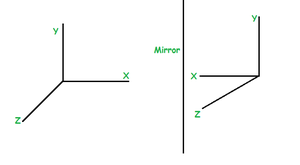Reflection along the Y-Z plane

The reflection transformation matrix for y-z axes is as follows:Consider, a point P[x, y, z] which is in 3D space is made to reflect along Y-Z direction, after reflection P[x, y, z] becomes P'[x’ ,y’ ,z’].3. Reflection along the X-Z plane: This is shown in the following figure –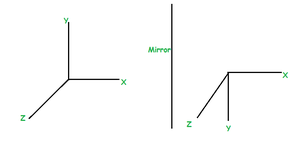Reflection along the X-Z plane:

The Reflection transformation matrix for z-x axes is as follows:Consider, a point P[x, y, z] which is in 3D space is made to reflect along Z-X direction, after reflection P[x,  y,  z] becomes P'[x’,  y’,  z’].Consider a cube ‘OABCDEFG’, which is given below, perform reflect transformation over it along X-Y plane.

The given cube is as follows: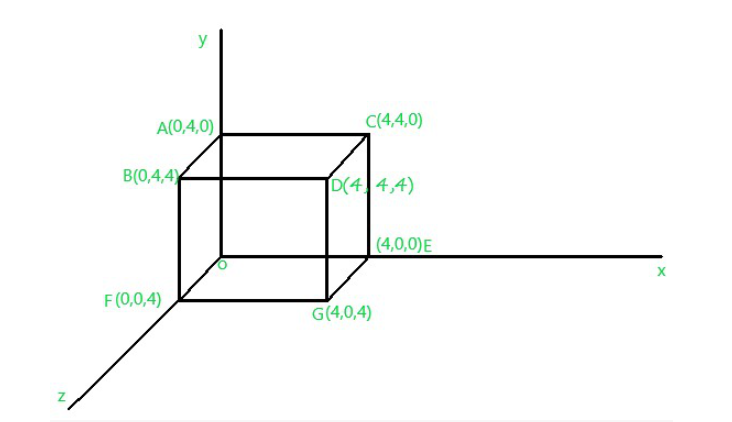Fig.1

So, Matrix representation condition of Reflection transformation along X-Y axis:Point O[0 0 0] becomes O’ after performing Reflection transformation:Point A[0 4 0] becomes A’ after performing Reflection transformation:Point B[0 4 4] becomes B’ after performing Reflection transformation:Point C[-4 4 0] becomes C’ after performing Reflection transformation:Point D[4 4 4] becomes D’ after performing Reflection transformation:Point E[4 0 0] becomes E’ after performing Reflection transformation:Point F[0 0 4] becomes F’ after performing Reflection transformation:Point G[4 0 4] becomes G’ after performing Reflection transformation:After performing Reflection Transformation over the above figure (Fig.1) would look like: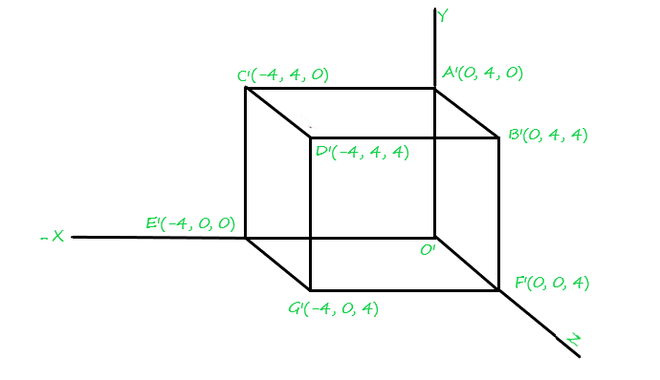Reflected 3D image

My Personal Notes arrow_drop_up